## The Problem

Guy has been measuring his refrigerator’s electricity consumption with a power meter. He wants to know if it’s using too much electricity and should be replaced, or if it’s running as expected.

The nameplate states that the unit will consume 296 kilowatt-hour (kWh) per year.

As we work through this problem, we should think carefully about variation, the measurement process, and the relation of the data to the specification. In real-world problems, getting these right is often the difference between success and failure.

For starters, we should ask what “296 kWh” means. Is it the design target or a projection based on some test? If it’s a target, is it an upper specification (“not to exceed”), a mean, or the median? Does it mean that some percentage of the time, this model of refrigerator will consume at most 296 kWh of electricity under given test conditions (a statistical upper limit), or that the refrigerator is flat-out defective if it ever exceeds 296 kWh in a year (a go/no-go condition such as used in manufacturing)?

In this case, we could probably find out with enough digging into European Union energy efficiency standards. Short of that kind of research, we can suppose that it’s either a mean or an upper limit. If the predicted electricity consumption is greater than this value, it’s time for Guy to consider replacing his refrigerator. Our statistical tests, then, should focus on the range of possible values and whether or not that range includes the nameplate rating.

## Setup

We’ll use the following libraries in this analysis.

library(tidyverse)
library(lubridate)
library(qcc)
library(ggrepel)
library(knitr)
library(kableExtra)
library(captioner)
library(RColorBrewer)
library(minpack.lm)
#library(gmodels)

## The Data

This is a fairly small dataset, so we can print it in its entirity.

Table 1: The data provided by Guy.
Date kWh Comment
16/06/18 0.00 NA
29/06/18 6.32 PC
01/07/18 7.97 NA
06/07/18 12.07 NA
08/07/18 13.90 NA
13/07/18 0.40 PC
15/07/18 2.09 NA
21/07/18 6.42 NA
22/07/18 7.77 NA
27/07/18 1.29 PC
03/08/18 6.73 NA
05/08/18 8.57 NA
10/08/18 12.42 NA

The power meter reports in cumulative kWh of electricity consumed. However, it resets every time there’s a power outage, and there have been several of those. The time of the power outages is not known, but the first measurement after a power outage is identified with a “PC” in the $$Comment$$ variable.

As with the specification, we need to be careful in thinking about this data. Guy’s measument process may be different than that intended by the manufacturer, and produce somewhat different results. That might not be a difference—a bias—of a factor of 2, but it could certainly be a bias of 20%.

Likewise, it’s likely that the refrigerator will consume electricity at different rates over time. Warmer or cooler room temperatures will certainly alter electricity consumption, as will more or less frequent access of the refrigerator and longer or shorter durations of access. A big party would probably bump that usage, too.

Likewise, the measurement process can introduce variaton. We have time-of-measurement in full day increments, but Guy may have recorded in the morning one day, the evening the next day, and the morning on the third day, so that even if the rate of electricity were constant, his observations would show an uneven day-to-day rate.

What this all means is that we need to use this small dataset for all that it’s worth, making sure that we data from a single population and taking full advantage of any variation we find in the data.

## EDA

The first step in any analysis is exploratory data analysis (EDA), where we examine the data’s structure, familiarize ourselves with its components, and use visualization to identify potential patterns.

Let us start with a first look at how the numeric data look. Since this is cumulative data over time, it makes sense to plot it as a simple time series against the row index.

# Preliminary EDA ##
rf_df %>%
mutate(index = row_number()) %>%
ggplot(aes(x = index, y = kWh)) +
geom_point() +
geom_label_repel(aes(label = Comment))
## Warning: Removed 10 rows containing missing values (geom_label_repel).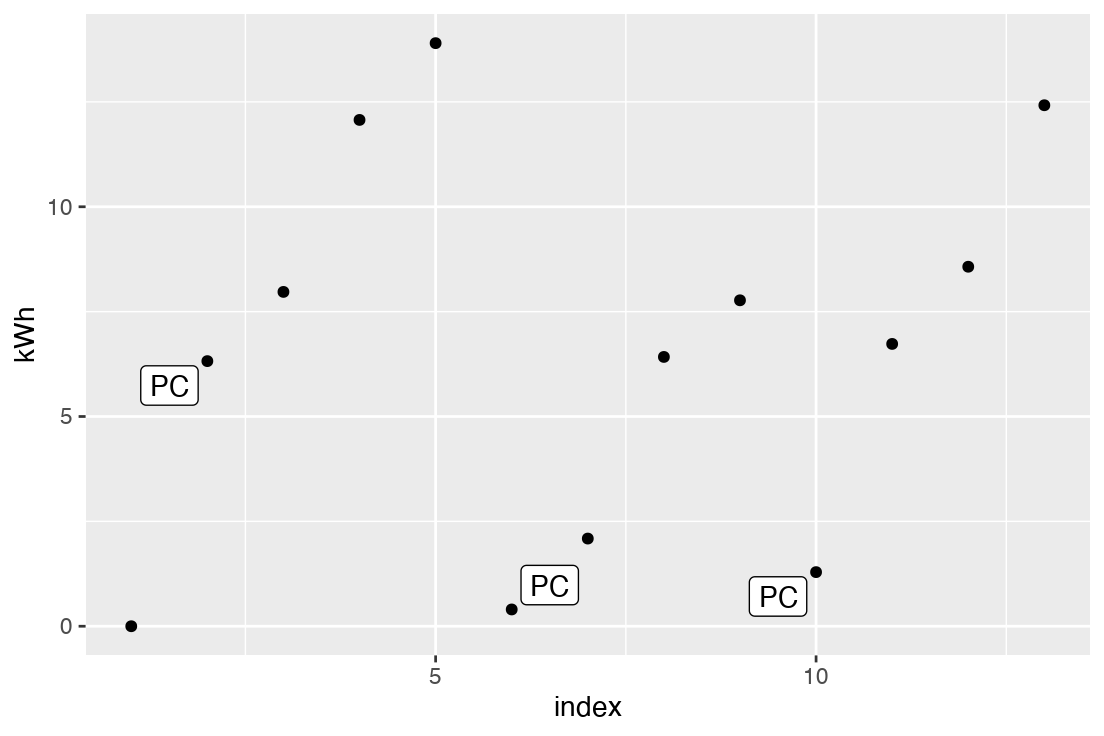Figure 1: Time series plot of the data.

The cumulative nature of the reported energy consumption, and the occasional resets, are both obvious.

It’s also appears that the consumption is not entirely linear—there is some variation in the rate of use from point to point. However, we’re looking at the data by row number rather than by date; maybe the apparent variation is just due to the irregularity of observations. After wrangling the data, we’ll look at this again.

Of note: the first data point precedes the first power outage. It seems unlikely that this will be of any use to us.

## Data wrangling

To predict annual electricity usage, we need to calculate a rate that we can then annualize. We can use the given cumulative numbers to derive an average daily rate between each observation. As we don’t know when each reset point was, we have to be careful about how we use these values. For instance, we want to eliminate negative rates (“drops” in cumulative kWh due to a reset), and we can’t make assumptions about when the power outages occur (i.e. we don’t know where the ’0’s are).

# Wrangle the data ##
# Fix the dates so we can do something useful
# Calculate the kWh used between each observation
# Calculate the days between observations
rf_df <- rf_df %>%
mutate(Date = dmy(Date)) %>%
mutate(diff = kWh - lag(kWh),
Date_Start = lag(Date)) %>%
mutate(days = Date - Date_Start) 

Now that we’ve fixed the $$Date$$ column, we can re-plot Figure 1 by date rather than by index.

rf_df %>%
ggplot(aes(x = Date, y = kWh)) +
geom_point()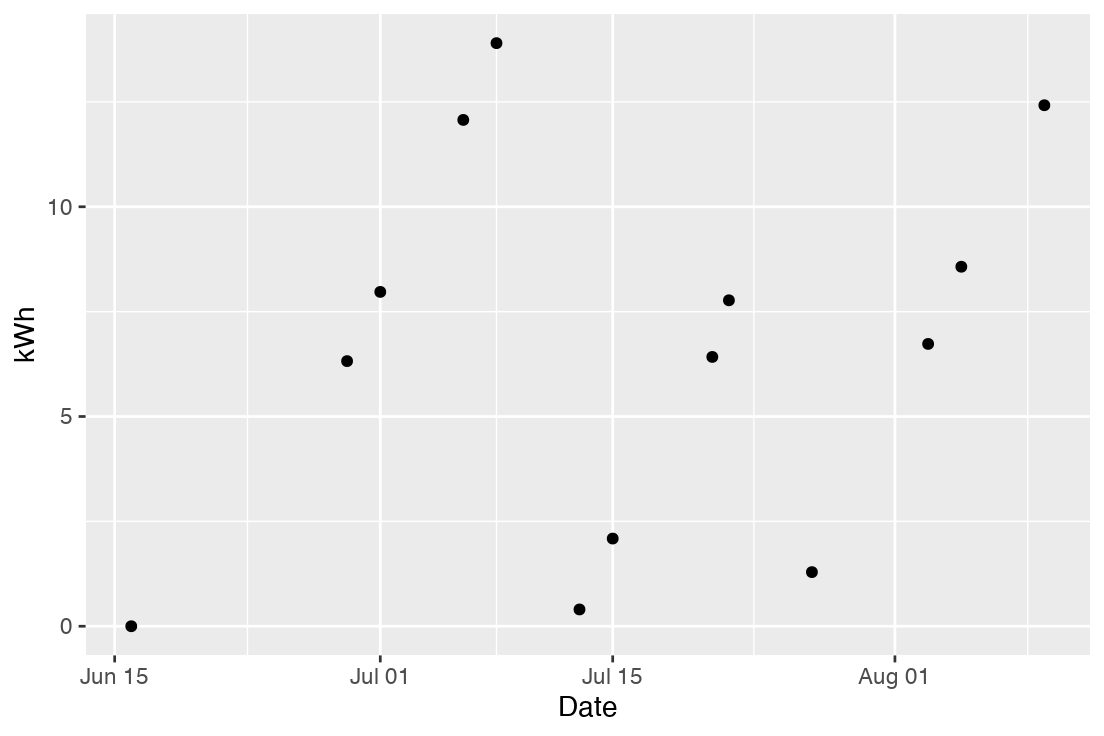Figure 3: Plot of cumulative electricity consumption in kWh versus date. Power outages and NA values have been removed.

The apparent variation in daily energy consumption may be a little less, plotted this way, but it’s still there. With a little more work, we might have made this a little more obvious by creating a grouping column and plotting a linear fit with geom_smooth(method = 'lm'), but for EDA that we’re not sharing, eyeballing it is good enough. Either the refrigerator is not constant in its electricity consumption (as we expected), or the power meter is inconsistent in measurement. Possibly both are sources of variation here.

We should also look at when measurements are collected, and this may tell us something.

daynames <- c('1' = "Monday",
'2' = "Tuesday",
'3' = "Wednesday",
'4' = "Thursday",
'5' = "Friday",
'6' = "Saturday",
'7' = "Sunday")
rf_df %>%
mutate(DOW = lubridate::wday(Date, week_start = 1)) %>%
group_by(DOW, Comment) %>%
summarise(n = n()) %>%
ungroup() %>%
mutate(DOW = as.factor(daynames[as.character(DOW)])) %>%
ggplot(aes(x = DOW, y = n, color = Comment)) +
geom_point() +
scale_x_discrete(breaks = daynames, label = daynames) +
labs(y = "Count of measurements",
x = "Day of measurement") +
coord_flip() +
facet_grid(.~Comment)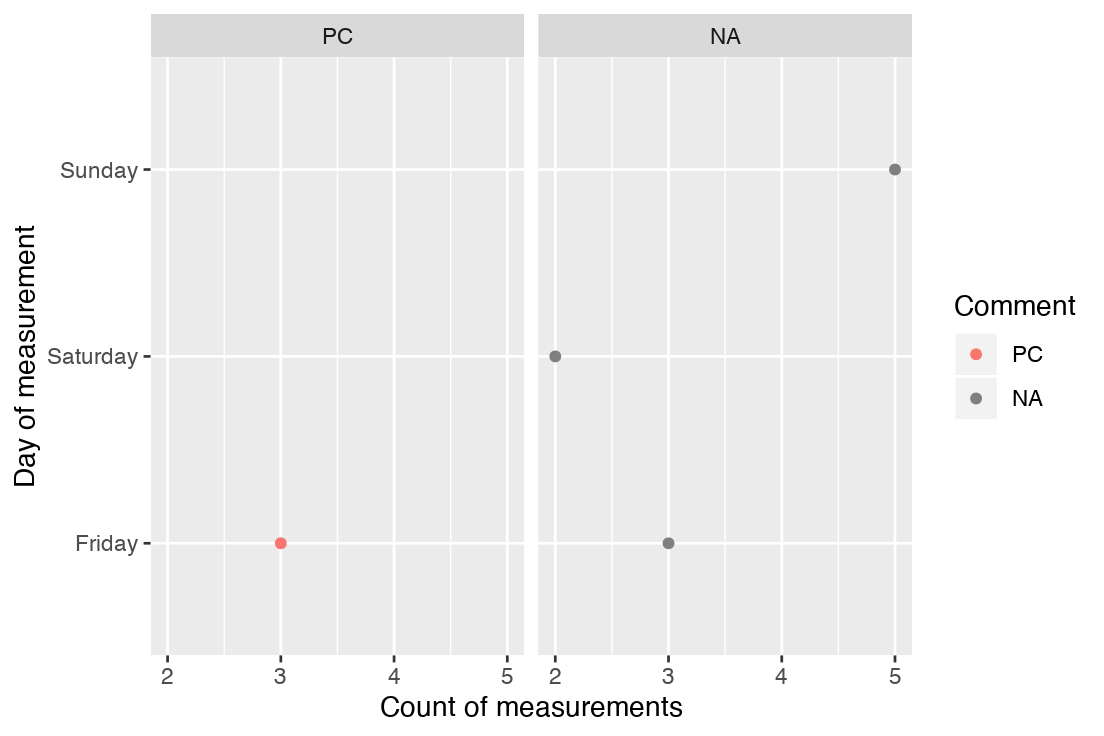We can see that all of the measurements have been taken on the weekend, and all of the power outages happened between Sunday and Friday.

If this is the case, we might expect Fridays to show higher consumption rates than Saturday or Sunday. We should calculate a rate for kWh consumption. We have to be careful, though, since those power outages throw off our totals.

# Due to power outages, lines with "PC" have invalid $diff values, # so convert to NA # Had some trouble doing this in dplyr due to the NAs... rf_df$diff[rf_df$Comment == "PC" & !is.na(rf_df$Comment)] <- NA

# Now drop the Comment column, and then drop any rows
# with NA values.
# This leaves us with useful observations of
# kWh used between dates.
# Now we calculate the average daily
# kWh consumption
rf_df <- rf_df %>%
select(-Comment) %>%
na.omit() %>%
mutate(daily_kWh = diff / as.integer(days))

Table 2 contains the updated, wrangled dataset.

Table 2: The data after wrangling.
Date kWh diff Date_Start days daily_kWh
2018-07-01 7.97 1.65 2018-06-29 NA 0.82
2018-07-06 12.07 4.10 2018-07-01 NA 0.82
2018-07-08 13.90 1.83 2018-07-06 NA 0.92
2018-07-15 2.09 1.69 2018-07-13 NA 0.84
2018-07-21 6.42 4.33 2018-07-15 NA 0.72
2018-07-22 7.77 1.35 2018-07-21 NA 1.35
2018-08-03 6.73 5.44 2018-07-27 NA 0.78
2018-08-05 8.57 1.84 2018-08-03 NA 0.92
2018-08-10 12.42 3.85 2018-08-05 NA 0.77

The key variable that we’re interested in is the average daily electricity use, $$daily\_kWh$$, so let’s plot it gainst the date of observation. Since this is an average over some number of days, it would be nice to also indicate over how many days each calculated value is averaged.

# Analysis ##
# Basic time series plot of the data
rf_df %>%
ggplot() +
geom_point(aes(x = Date, y = daily_kWh)) +
geom_segment(aes(x = Date_Start, y = daily_kWh, xend = Date, yend = daily_kWh)) +
labs(x = "Date",
y = "Average Daily Consumption, kWh")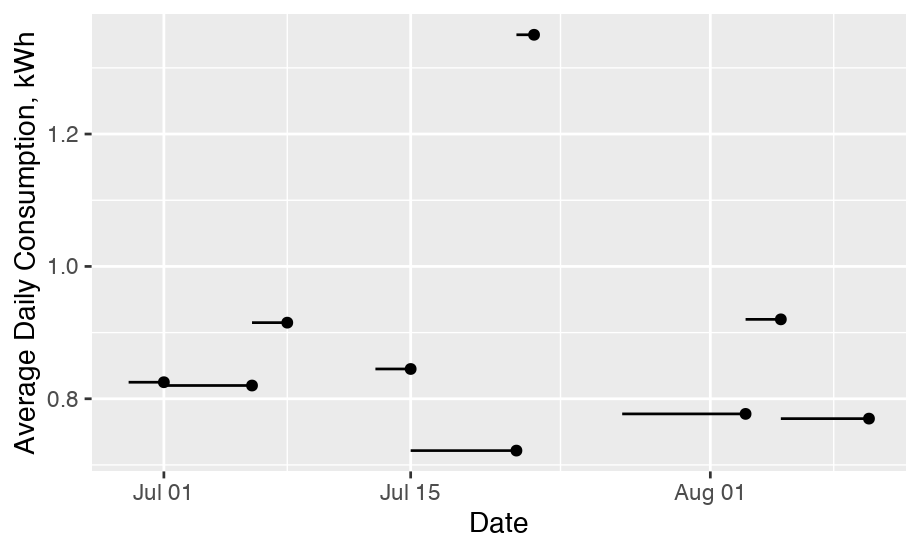Figure 4: The average daily kWh used by date. Lines represent the span of days over which the average is taken.

There only obvious pattern that I’m picking up here is that cumulative readings over more days may be showing lower rates of daily consumption.

rf_df %>%
mutate(days = as.integer(days)) %>%
ggplot(aes(x = days, y = daily_kWh)) +
geom_point()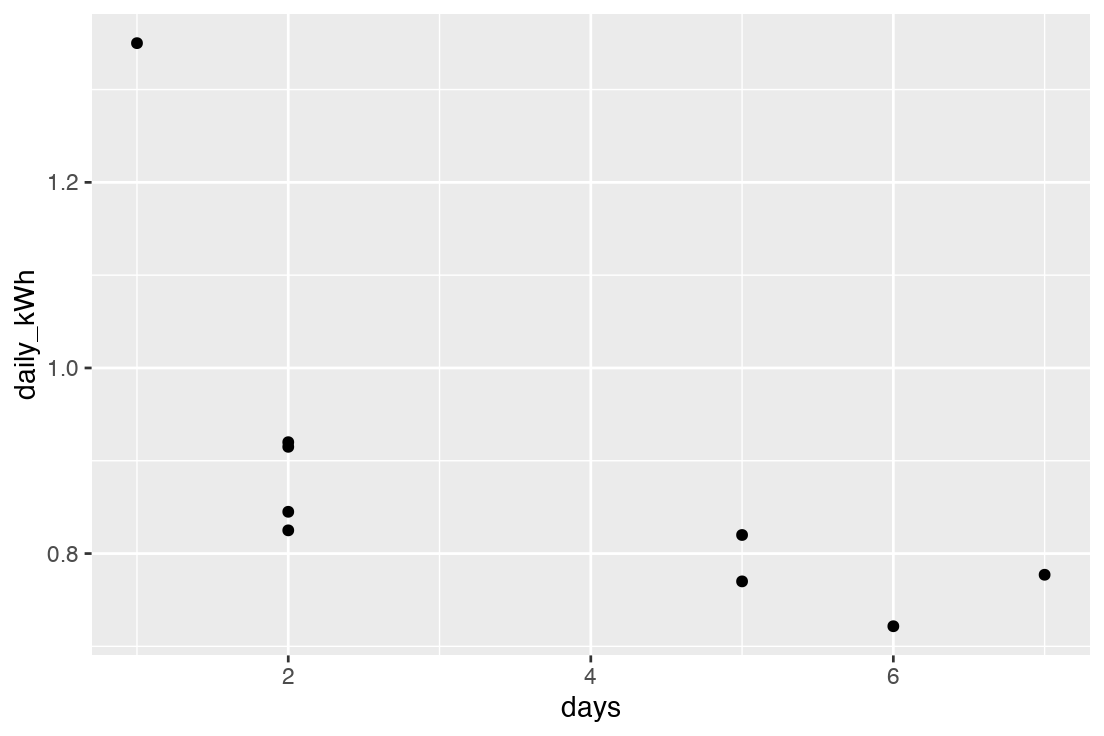We might have expected lower average daily consumption over longer observation intervals. First, longer observation intervals will tend toward the distribution mean, rather than the high rates of consumption that we might expect over shorter periods (see below for more on the long tail). Additionally, longer intervals (larger values of $$days$$) may indicate periods where the refrigerator was not opened, and therefore operating at minimal power draw, while shorter periods could indicate heavy use.

Beyond that, there is not a general upward trend, or trend toward longer or shorter observation intervals.

Now that we have values that are directly comparable in the $$daily\_kWh$$ variable, we should also look at its distribution, as this might tell us something useful about the assumptions that we can make during our analysis.

rf_df %>%
ggplot(aes(x = daily_kWh)) +
geom_histogram(bins = 10)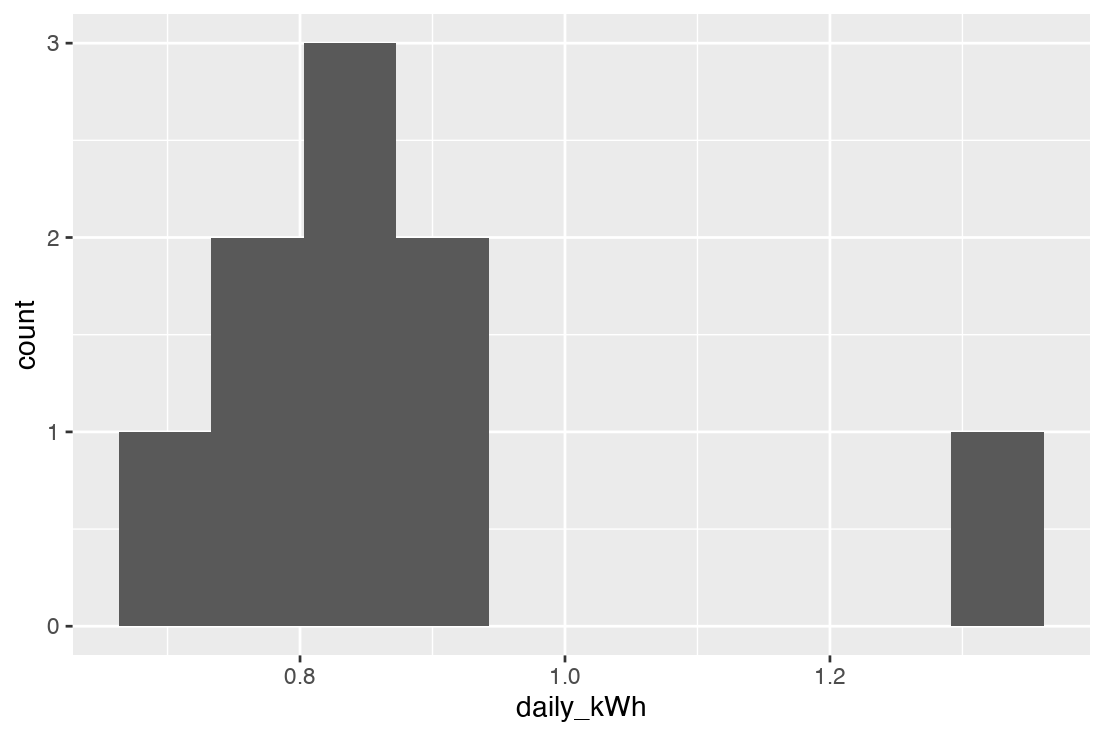Figure 2: Distribution of the calculated daily electricity use.

We appear to have a skewed distribution with a long tail toward higher values, or possibly two separate distributions (i.e. this may be a bimodal distribution) with one distribution centered near 0.8 and the other somewhere above 1.2 kWh/day.

We might expect to see two distributions if there are really two processes at play.

At the same time, with only common-cause variation in usage and measurement, we would expect to see high days and low days. While the low days must be bound by 0 kWh/day, the upper bound is determined only by the maximum power use of the compressor and lights. The distribution of daily kWh should be skewed with a long tail toward higher numbers, and we could be seeing that here.

We should also have a look at the days when measurements were taken (thanks to Guy for this suggestion), to see if there’s anything interesting or suggestive in the data.

rf_df %>%
mutate(DOW = lubridate::wday(Date, week_start = 1)) %>%
group_by(DOW) %>%
summarise(mean_daily_kWh = mean(daily_kWh),
n = n(),
sd = sd(daily_kWh)) %>%
mutate(se = sd / sqrt(n),
ci_lo = mean_daily_kWh - qt(1 - (0.05 / 2), n - 1) * se,
ci_hi = mean_daily_kWh + qt(1 - (0.05 / 2), n - 1) * se) %>%
# mutate(conf_lo = gmodels::ci(daily_kWh),
#        conf_hi = ci(daily_kWh)) %>%
ungroup() %>%
mutate(DOW = as.factor(daynames[as.character(DOW)])) %>%
kable(format = "html", digits = 2) %>%
kable_styling(bootstrap_options = c("striped", "condensed"),
full_width = FALSE)
DOW mean_daily_kWh n sd se ci_lo ci_hi
Friday 0.79 3 0.03 0.02 0.72 0.86
Saturday 0.72 1 NA NA NaN NaN
Sunday 0.97 5 0.22 0.10 0.70 1.24

With only 9 datapoints, it’s hard to gain much information from this. Friday and Saturday seem to be similar, while Sunday might be a little higher. If this house were unused during the week, we might have expected that Friday would be much lower than either Saturday or Sunday. However, with confidence intervals spanning all three day’s mean readings, there is no significant difference between them, and we cannot learn anything further.

## Analysis and Results

Our analysis will first check that the data is suitable for making predictions. We will then predict a range of likely annual values, and finally assess whether the specification of 296 kWh per annum falls with those predictions.

### Data homogeneity

In Figure 4 we were left wondering if we have skewed data from one process or symmetric data from two processes.

This question is critical to our ability to reliably predict future data. We have sampled some process, and in order to make predictions about the future, we need those samples to all come from a singular, or homogeneous, process. If we’re seeing samples from different, or heterogeneous, processes then we either have to segregate the data or give up making valid predictions.(Wheeler 2009a)

We can use an individuals and moving range (“ImR”) chart to quickly assess whether this data exhibits homogeneous properties—are we sampling from a single population of data and can make predictions about the future?

# Use qcc to plot the individual values and the moving range
rf_daily_sd <- rf_r$center We have an average daily consumption of 0.82 kWh, with a standard deviation of 0.20 kWh. #### Simulating a year, over and over With these population parameter estimates, we use Monte Carlo simulation to estimate annual electricity use for 1000 identical cases. We do this by simulating daily consumption for 365 days and adding to obtain an annual figure. We then repeat this process 1000 times. # Use Monte Carlo simulation with these values to simulate # 1000 identical refrigerators operating for a year num_replicates <- 10000 rf_annual <- replicate(expr = rnorm(n = 365, mean = rf_daily_mean, sd = rf_daily_sd) %>% sum(), n = num_replicates) head(rf_annual) ##  294.5972 296.1955 292.1577 294.8222 292.8706 301.2250 # Population mean and standard deviation, based on the moving range rf_annual_mean <- mean(rf_annual) rf_annual_sd <- sd(rf_annual) We can compare the simulated annual performance to the nameplate performance with a histogram. rf_annual_df <- tibble(annual_total_kWh = rf_annual) rf_annual_df %>% ggplot(aes(x = annual_total_kWh)) + geom_histogram(bins = 17, aes(y = ..density..)) + geom_vline(xintercept = 296, color = "red") + labs(x = "Annual electricity use, kWh") + theme_linedraw() + theme(axis.title.y = element_blank(), axis.text.y = element_blank(), axis.ticks.y = element_blank())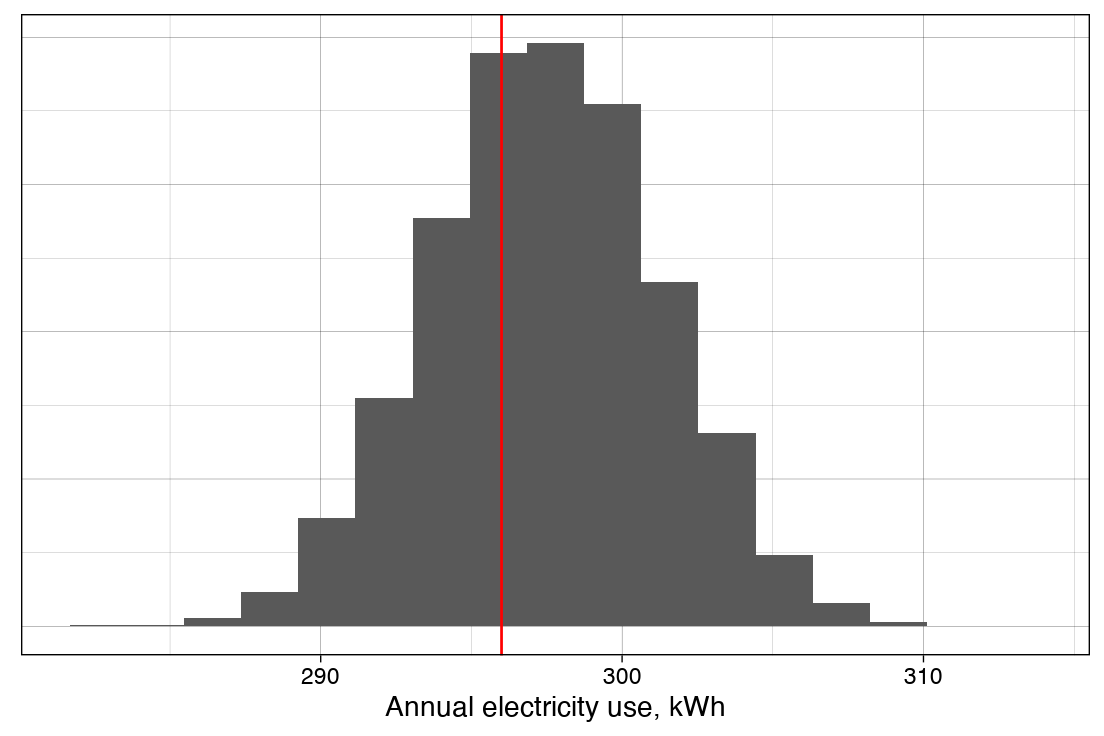Figure 7: Range of likely annual consumption predicted using a normal distribution and population parameters from the ImR charts. Red line indicates nameplate annual consumption. This looks like the refrigerator is at least operating in the range of the nameplate electricity consumption. It appears to be normally distributed, as we expected from the CLT. We can test that with a test for non-normality like the Anderson-Darling test. nortest::ad.test(rf_annual_df$annual_total_kWh)
##
##  Anderson-Darling normality test
##
## data:  rf_annual_df$annual_total_kWh ## A = 0.23438, p-value = 0.794 And it does, as expected, fail to reject the null hypothesis in the lack of fit test. ### Bootstrap #### Bootstrapping with Monte Carlo simulation To bootstrap, we use the samples that we have to create larger data sets. This is accomplished by randomly resampling with replacement from the existing samples. Unlike parametric methods (e.g. assuming a normal distribution), bootstrapping preserves characteristics of the sample distribution—which presumably mirrors the population distribution—while still allowing us to estimate population parameters such as mean, standard deviation, and confidence intervals, and to perform other statistical analyses such as regression. In this case, we’ll bootstrap a year’s worth of daily consumption from our observed samples. We can then add up the daily values to obtain an estimate for the year, or estimate population statististics for the daily values. One bootstrap provides us with a single estimate. As with the previous method, we use Monte Carlo simulation to repeat the bootstrap many times in order to estimate population parameters like mean and standard deviation. # Calculate a weight for sampling rf_df <- rf_df %>% mutate(weight = as.integer(days) / sum(as.integer(days))) # Set up a data frame to store results bs_annual_df <- tibble(annual_total_kWh = rep(NA, times = num_replicates), daily_avg_kWh = rep(NA, times = num_replicates), daily_sd_kWh = rep(NA, times = num_replicates)) # Monte Carlo simulation num_replicates times for(i in 1:num_replicates) { # Bootstrap for a year's worth of daily values bs_annual <- sample(x = rf_df$daily_kWh,
size = 365,
replace = TRUE,
prob = rf_df$weight) # Calculate and store the cumulative total, daily mean, and daily std dev bs_annual_df[i, "annual_total_kWh"] <- sum(bs_annual) bs_annual_df[i, "daily_avg_kWh"] <- mean(bs_annual) bs_annual_df[i, "daily_sd_kWh"] <- sd(bs_annual) } #### Examining the bootstrap Having obtained our 10 000 annual estimates, we can now see how they are distributed and compare them to the refrigerator’s nameplate consumption, as we did for the normal distribution-based analysis in Figure 7: Range of likely annual consumption predicted using a normal distribution and population parameters from the ImR charts. Red line indicates nameplate annual consumption.. # Plot annual estimates and test for normality bs_annual_df %>% ggplot(aes(x = annual_total_kWh)) + geom_histogram(bins = 25, aes(y = ..density..)) + geom_vline(xintercept = 296, color = "red") + labs(x = "Annual electricity use, kWh") + scale_x_continuous(limits = range(rf_annual_df$annual_total_kWh)) +
theme_linedraw() +
theme(axis.title.y = element_blank(),
axis.text.y = element_blank(),
axis.ticks.y = element_blank())